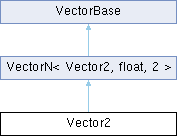MaterialX 1.38.8
Searching...
No Matches
Vector2 Class Reference

A vector of two floating-point values. More...

`#include <Types.h>`

Inheritance diagram for Vector2:## Public Member Functions

Vector2 (float x, float y)

float cross (const Vector2 &rhs) const
Return the cross product of two vectors.Public Member Functions inherited from VectorN< Vector2, float, 2 >
VectorN (Uninit)

VectorN (float s)

VectorN (const std::array< float, N > &arr)

VectorN (const vector< float > &vec)

VectorN (const float *begin, const float *end)

bool operator== (const Vector2 &rhs) const
Return true if the given vector is identical to this one.

bool operator!= (const Vector2 &rhs) const
Return true if the given vector differs from this one.

bool operator< (const Vector2 &rhs) const
Compare two vectors lexicographically.

float & operator[] (size_t i)
Return the scalar value at the given index.

const float & operator[] (size_t i) const
Return the const scalar value at the given index.

Vector2 operator+ (const Vector2 &rhs) const

VectorNoperator+= (const Vector2 &rhs)

Vector2 operator- (const Vector2 &rhs) const
Component-wise subtraction of two vectors.

Vector2 operator- () const
Unary negation of a vector.

VectorNoperator-= (const Vector2 &rhs)
Component-wise subtraction of two vectors.

Vector2 operator* (const Vector2 &rhs) const
Component-wise multiplication of two vectors.

Vector2 operator* (float s) const
Component-wise multiplication of a vector by a scalar.

VectorNoperator*= (const Vector2 &rhs)
Component-wise multiplication of two vectors.

VectorNoperator*= (float s)
Component-wise multiplication of a vector by a scalar.

Vector2 operator/ (const Vector2 &rhs) const
Component-wise division of two vectors.

Vector2 operator/ (float s) const
Component-wise division of a vector by a scalar.

VectorNoperator/= (const Vector2 &rhs)
Component-wise division of two vectors.

VectorNoperator/= (float s)
Component-wise division of a vector by a scalar.

float getMagnitude () const
Return the magnitude of the vector.

Vector2 getNormalized () const
Return a normalized vector.

float dot (const Vector2 &rhs) const
Return the dot product of two vectors.

Iterator begin ()

ConstIterator begin () const

Iterator end ()

ConstIterator end () const

float * data ()
Return a pointer to the underlying data array.

const float * data () const
Return a const pointer to the underlying data array.Public Types inherited from VectorN< Vector2, float, 2 >
using Iterator = typename std::array< float, N >::iterator

using ConstIterator = typename std::array< float, N >::const_iteratorStatic Public Member Functions inherited from VectorN< Vector2, float, 2 >
static constexpr size_t numElements ()
Return the number of scalar elements for the vector.Protected Attributes inherited from VectorN< Vector2, float, 2 >
std::array< float, N > _arr

## Detailed Description

A vector of two floating-point values.

The documentation for this class was generated from the following file: